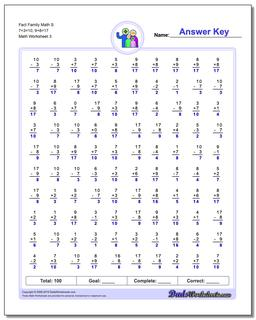# Math Worksheets: Fact Family Math: Fact Family Math: Fact Family Math S 7+3=10, 9+8=17 (Third Worksheet)## Fact Family Math S 7+3=10, 9+8=17 (Third Worksheet)

PropertyValue
DescriptionFact Family Math S 7+3=10, 9+8=17: These math worksheets have 100 addition and subtraction fact family problems and make for a challenging two minute test. (Third Worksheet)
Resource TypeWorksheet